What does it do?

Sorts a table based on a column and order specified

Formula breakdown:

=SORT(array, [sort_index], [sort_order])

What it means:

=SORT(data to be sorted, [which column to be used for sorting], [ascending or descending order])

Did you know that you can now sort your table data with an Excel Formula? Yes you can! It is definitely possible now with Excel’s SORT Formula. It is a new formula introduced in Office 365 released in 2018!

We have a tax table that we want to sort by the tax rate in a descending order.

I explain how you can do this below:STEP 1: We need to enter the SORT function in a blank cell:

## =SORT(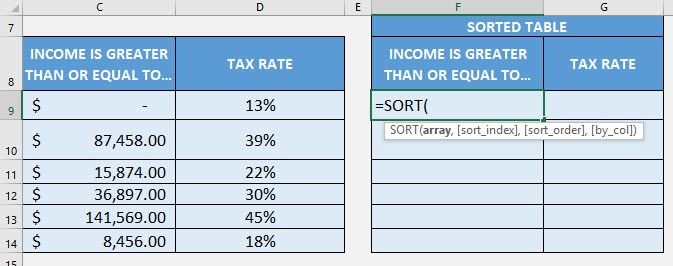STEP 2: The SORT arguments:

## array

What is the data to be sorted?

Select the cells containing the tax data, do not include the headers:

## =SORT(C9:D14,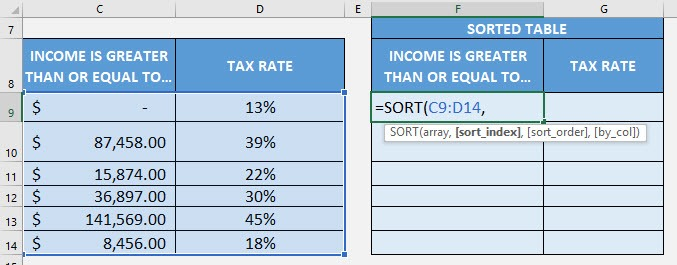## [sort_index]

What is the column to be used for sorting?

We specify the column number here. Since the tax rate column is the second column, place in 2.

## =SORT(C9:D14, 2,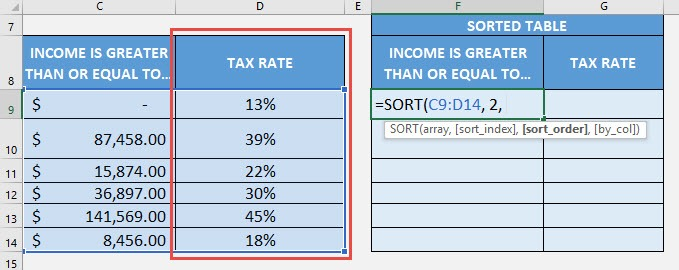## [sort_order]

What is the sort order? 1 for Ascending, -1 for Descending order.

Since we want descending order, place in -1.

## =SORT(C9:D14, 2, -1)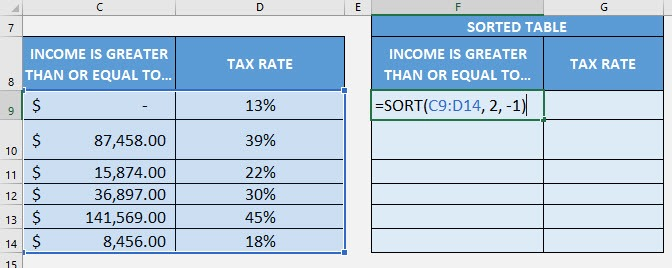Now it gets sorted magically!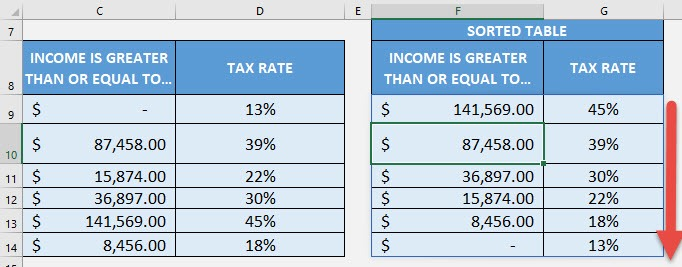How to Use the SORT Formula in Excel